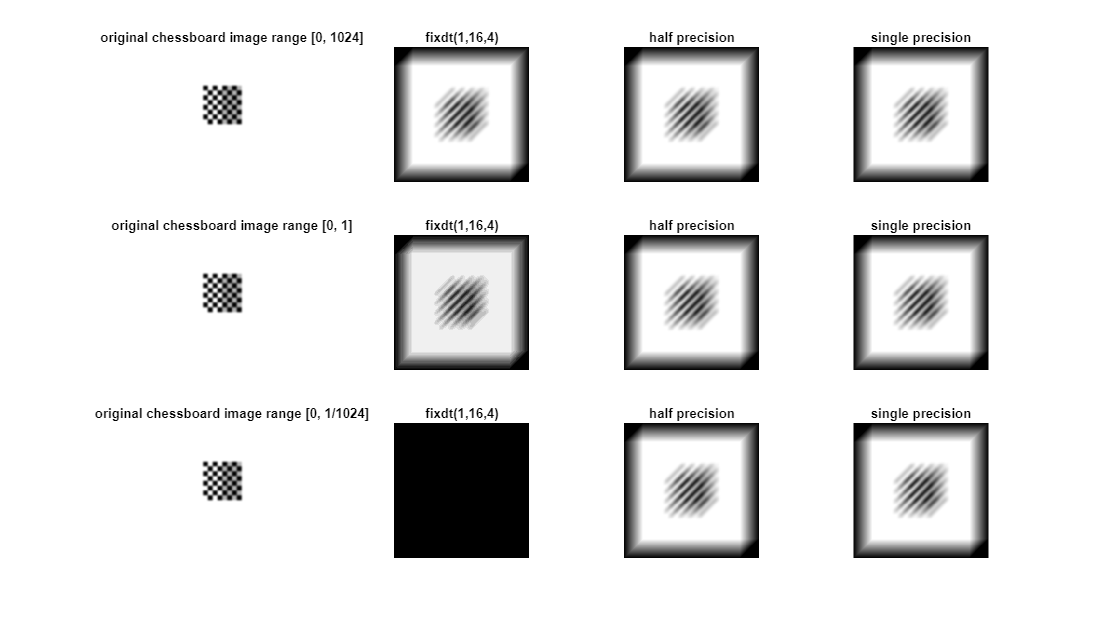# Image Quantization with Half-Precision Data Types

This example shows the effects of quantization on images. The `ex_imagequantization` model, computes the two-dimensional fourier transform of an image of a checkerboard. The original image is displayed in the left-most column, and the result is displayed with fixed-point, half-precision, and single-precision data types. You can see in the resulting images that, while the fixed-point data type does not always produce an acceptable result, the half-precision data type, which uses the same number of bits as the fixed-point data type, produces a result comparable to the single-precision result.

```model = 'ex_image_quantization.slx'; open_system(model); sim(model)```## Support평가판 신청